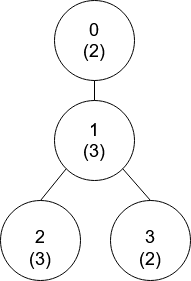Formatted question description: https://leetcode.ca/all/1766.html

# 1766. Tree of Coprimes

Hard

## Description

There is a tree (i.e., a connected, undirected graph that has no cycles) consisting of n nodes numbered from 0 to n - 1 and exactly n - 1 edges. Each node has a value associated with it, and the root of the tree is node 0.

To represent this tree, you are given an integer array nums and a 2D array edges. Each nums[i] represents the i-th node’s value, and each edges[j] = [u_j, v_j] represents an edge between nodes u_j and v_j in the tree.

Two values x and y are coprime if gcd(x, y) == 1 where gcd(x, y) is the greatest common divisor of x and y.

An ancestor of a node i is any other node on the shortest path from node i to the root. A node is not considered an ancestor of itself.

Return an array ans of size n, where ans[i] is the closest ancestor to node i such that nums[i] and nums[ans[i]] are coprime, or -1 if there is no such ancestor.

Example 1:Input: nums = [2,3,3,2], edges = [[0,1],[1,2],[1,3]]

Output: [-1,0,0,1]

Explanation: In the above figure, each node’s value is in parentheses.

• Node 0 has no coprime ancestors.
• Node 1 has only one ancestor, node 0. Their values are coprime (gcd(2,3) == 1).
• Node 2 has two ancestors, nodes 1 and 0. Node 1’s value is not coprime (gcd(3,3) == 3), but node 0’s value is (gcd(2,3) == 1), so node 0 is the closest valid ancestor.
• Node 3 has two ancestors, nodes 1 and 0. It is coprime with node 1 (gcd(3,2) == 1), so node 1 is its closest valid ancestor.

Example 2:Input: nums = [5,6,10,2,3,6,15], edges = [[0,1],[0,2],[1,3],[1,4],[2,5],[2,6]]

Output: [-1,0,-1,0,0,0,-1]

Constraints:

• nums.length == n
• 1 <= nums[i] <= 50
• 1 <= n <= 10^5
• edges.length == n - 1
• edges[j].length == 2
• 0 <= u_j, v_j < n
• u_j != v_j

## Solution

First, store each node’s adjacent nodes. Next, preprocess the numbers from 1 to 50 and find coprimes for each number. Then, do depth first search starting from root node 0 and fimd the nearest coprime node for each node.

class Solution {
int[] ans;
Map<Integer, List<Integer>> edgesMap = new HashMap<Integer, List<Integer>>();
Map<Integer, List<Integer>> coprimesMap = new HashMap<Integer, List<Integer>>();
int[] depths;
int[] pos = new int;

public int[] getCoprimes(int[] nums, int[][] edges) {
int n = nums.length;
ans = new int[n];
depths = new int[n];
Arrays.fill(ans, -1);
Arrays.fill(pos, -1);
for (int[] edge : edges) {
int node0 = edge, node1 = edge;
List<Integer> list0 = edgesMap.getOrDefault(node0, new ArrayList<Integer>());
List<Integer> list1 = edgesMap.getOrDefault(node1, new ArrayList<Integer>());
edgesMap.put(node0, list0);
edgesMap.put(node1, list1);
}
for (int i = 1; i <= 50; i++) {
for (int j = 1; j <= 50; j++) {
if (gcd(i, j) == 1) {
List<Integer> list = coprimesMap.getOrDefault(i, new ArrayList<Integer>());
coprimesMap.put(i, list);
}
}
}
depthFirstSearch(nums, 0, -1);
return ans;
}

public void depthFirstSearch(int[] nums, int u, int form) {
int t = nums[u];
for (int v : coprimesMap.get(t)) {
if (pos[v] != -1) {
if (ans[u] == -1 || depths[ans[u]] < depths[pos[v]])
ans[u] = pos[v];
}
}
int p = pos[t];
pos[t] = u;
for (int i : edgesMap.get(u)) {
if (i != form) {
depths[i] = depths[u] + 1;
depthFirstSearch(nums, i, u);
}
}
pos[t] = p;
}

public int gcd(int a, int b) {
while (a != 0 && b != 0) {
if (a >= b)
a %= b;
else
b %= a;
}
return a == 0 ? b : a;
}
}